Dirichlet integral

A functional connected with the solution of the Dirichlet problem for the Laplace equation by the variational method. Letbe a bounded domain inwith boundaryof class, let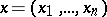and let the function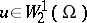(cf. Sobolev space). The Dirichlet integral for the functionis the expression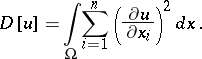For a certain given functiononone considers the setof functions fromwhich satisfy the boundary condition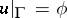. If the set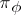is non-empty, there exists a unique function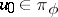for whichand this function is harmonic in. The converse theorem is also true: If a harmonic functionbelongs to the set, thenis attained on it. Thus,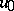is a generalized solution fromof the Dirichlet problem for the Laplace equation. However, not for every functionit is possible to find a function. There exists even continuous functions onfor which the setis empty, i.e. the space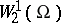contains no functionssatisfying the condition. The classical solution of the Dirichlet problem for the Laplace equation with such boundary functioncannot have a finite Dirichlet integral and is not a generalized solution from the space.